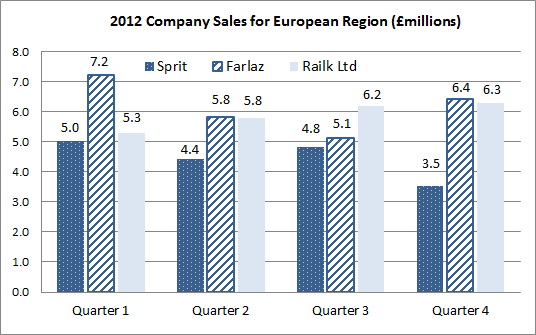## Classic Question 1: By what percent would Sprit’s fourth quarter 2012 sales have to increase in order to equal its third quarter sales figures?Step 1: find the right numbers from the chart.

Make sure you are comparing the right numbers. This means reading and understanding the question. We are looking at the increase required to get from 3.5 (£million ) to 4.8 (£million). We’ll ignore the £million since the units have no effect on the increase.

Step 2: Recast the equation to solve.

The problem boils down to: 3.5 x ( ? ) = 4.8. This can be recast as:

? = (4.8 ÷ 3.5)

? = 1.371

Ans = 37.1% increase

Whilst you probably won’t have time to do this in your real numerical reasoning test, for our training it is worth double-checking that this answer is correct. To do this we say is 3.5 x 1.371 = 4.8? Putting this into the calculator we see that yes, this works!